# Basic Plasma Parameters

Previous Page  – Brief history of plasmas

This page is accompanied with a short video explaining basic plasma paramters.

Consider an idealized plasma consisting of an equal number of electrons, with massand charge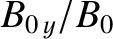(here,denotes the magnitude of the electron charge), and ions, with mass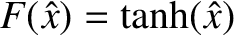and charge. We do not necessarily demand that the system has attained thermal equilibrium, but nevertheless use the symbol(1)

to denote a kinetic temperature measured in energy units (i.e., joules). Here,is a particle speed, and the angular brackets denote an ensemble average. The kinetic temperature of speciesis essentially the average kinetic energy of particles of this species. In plasma physics, kinetic temperature is invariably measured in electron-volts (1 joule is equivalent toeV).

Quasi-neutrality demands that(2)

whereis the number density (i.e., the number of particles per cubic meter) of species.

Assuming that both ions and electrons are characterized by the same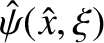(which is, by no means, always the case in plasmas), we can estimate typical particle speeds via the so-called thermal speed,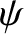(3)

Note that the ion thermal speed is usually far smaller than the electron thermal speed: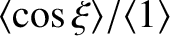(4)

Of course,andare generally functions of position in a plasma.

This site uses Akismet to reduce spam. Learn how your comment data is processed.